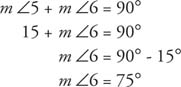## Special Angles

Certain angle pairs are given special names based on their relative position to one another or based on the sum of their respective measures.

Adjacent angles are any two angles that share a common side separating the two angles and that share a common vertex. In Figure 1, ∠1 and ∠2 are adjacent angles.## Vertical angles

Vertical angles are formed when two lines intersect and form four angles. Any two of these angles that are not adjacent angles are called vertical angles. In Figure 2, line l and line m intersect at point Q, forming ∠1, ∠2, ∠3, and ∠4.Figure 2 Two pairs of vertical angles and four pairs of adjacent angles.

• Vertical angles:
• ∠1 and ∠3
• ∠2 and ∠4
• ∠1 and ∠2
• ∠2 and ∠3
• ∠3 and ∠4
• ∠4 and ∠1

Theorem 7: Vertical angles are equal in measure.

## Complementary angles

Complementary angles are any two angles whose sum is 90°. In Figure 3, because ∠ ABC is a right angle, m ∠1 + m ∠2 = 90°, so ∠1 and ∠2 are complementary.Complementary angles do not need to be adjacent. In Figure 4, because m ∠3 + m ∠4 = 90°, ∠3, and ∠4, are complementary.Example 1: If ∠5 and ∠6 are complementary, and m ∠5 = 15°, find m ∠6.

Because ∠5 and ∠6 are complementary,Theorem 8: If two angles are complementary to the same angle, or to equal angles, then they are equal to each other.

Refer to Figures 5 and 6. In Figure 5, ∠ A and ∠ B are complementary. Also, ∠ C and ∠ B are complementary. Theorem 8 tells you that mA = mC. In Figure 6, ∠ A and ∠ B are complementary. Also, ∠ C and ∠ D are complementary, and mB = mD. Theorem 8 now tells you that mA = mC.Figure 5 Two angles complementary to the same angleFigure 6 Two angles complementary to equal angles

Supplementary angles

Supplementary angles are two angles whose sum is 180°. In Figure , ∠ ABC is a straight angle. Therefore m ∠6 + m ∠7 = 180°, so ∠6 and ∠7 are supplementary.Theorem 9: If two adjacent angles have their noncommon sides lying on a line, then they are supplementary angles.

Supplementary angles do not need to be adjacent (Figure 8).